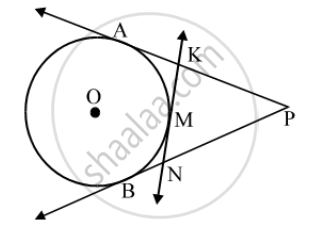# PA and PB are tangents from P to the circle with centre O. At point M, a tangent is drawn cutting PA at K and PB at N. Prove that KN = AK + BN. - Mathematics

Sum

PA and PB are tangents from P to the circle with centre O. At point M, a tangent is drawn cutting PA at K and PB at N. Prove that KN = AK + BN.

#### Solution

We know that the tangents drawn from an external point to a circle are equal in length.∴ PA = PB …. (i) [From P]

KA = KM …. (ii) [From K] and, NB = NM …. (iii) [From N]

Adding equations (ii) and (iii), we get

KA + NB = KM + NM

⇒ AK + BN = KM + MN ⇒ AK + BN = KN

Concept: Concept of Circle - Centre, Radius, Diameter, Arc, Sector, Chord, Segment, Semicircle, Circumference, Interior and Exterior, Concentric Circles
Is there an error in this question or solution?
Share